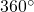# 5.5 Four Shapes and the Period

Here are the four basic shapes of a sinusoidal curve: positive and negative sine; positive and negative cosine.

The period of the curve is the distance along the horizontal axis between one point on the curve and the same point on the next wave – eg, from crest to crest or from trough to trough.

The period informs us by how much the curve has been stretched horizontally.

Consider the value. The period of the curveis.

Use the applet below to see the effect of the parameterwhenIn general, once the period has been identified the value b can be calculated as follows:alternatively,Identify the function in the following 12 graphs: